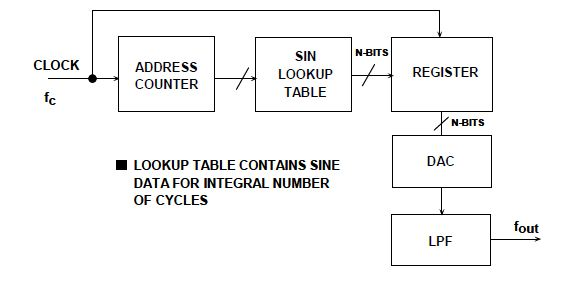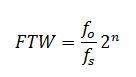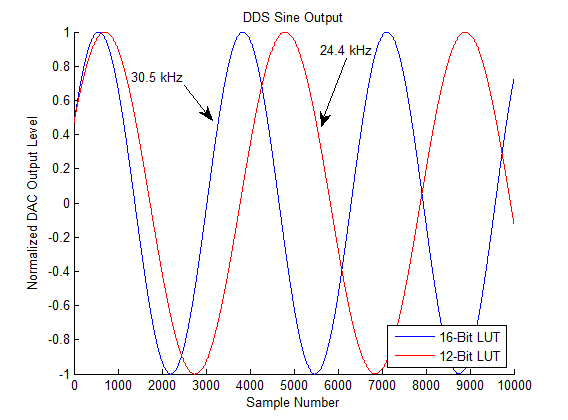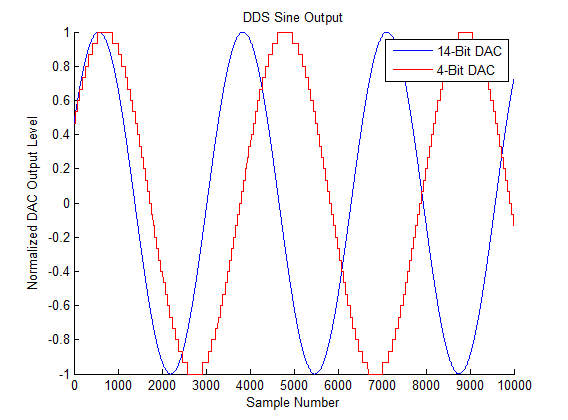# Modeling a DDS in MATLAB

Posted by in on 2-21-13

Over the last few decades, we’ve seen the analog components of electronic systems slowly shrink as digital electronics take over that space. One important component driving that evolution is the direct digital synthesizer (DDS) chip.

Because the DDS is not an analog device, there are some nuances derived from the quantized digital implementation that need to be considered in a design. For this, and maybe hundreds of other reasons, you may want to simulate or model a DDS in MATLAB. This article gives a brief overview on how a DDS works and demonstrates an idealized model in MATLAB.

1. DDS Concept Overview
2. MATLAB DDS Model
3. M-Code Discussion
4. Other Modeling Considerations

## DDS Concept Overview

The operation of direct digital synthesizer (DDS) can be simply generalized as a memory chip connected to a digital to analog converter (DAC). A very simplified block diagram is shown below.The phase of a single cycle sine wave (from 0 to 2*pi) is divided into 2^n divisions. That single cycle sine wave is stored in read-only memory, or a lookup table (LUT), and the desired sinusoid phase is used as an address to that LUT.

The frequency at which the address of the LUT changes is directly proportional to the frequency of the resulting sine wave.

Additionally, if some of the addresses of the phase LUT are skipped, the frequency of the resulting sine wave is also increases. For example, addressing every other position of the LUT will produce a sinusoid of twice the frequency of addressing every position of the sine LUT.

Because the phase of the sinusoid must be represented by a fixed-point number, the phase and frequency is quantized. The number of LUT addresses that are skipped on every clock cycle to obtain a certain frequency is called a frequency tuning word (FTW). Because the FTW must be an integer value (how could you address LUT position 1.69?), the rate of change of the phase is quantized. Frequency is defined as the rate of change in phase, so clearly the frequency is also quantized.

Because of phase quantization, for a given clock frequency, there are certain frequencies that cannot be exactly achieved. In the example later in this article, you’ll see that a desired frequency of 30 MHz cannot be achieved, and a 24.4 MHz sinusoid is produced.

The DAC precision (or number of bits) predictable quantizes the output sine wave, with higher precision DACs producing smoother sinusoids.

More details on DDS architecture, including operational equations, can be found in this white paper published by Analog Devices.

## MATLAB DDS

[cc lang=”matlab” noborder=”true” tab_size=”4″ lines=”-1″ width=”600″ escaped=”true”]
function [s]=ideal_dds(fs,bp,bd,fd,ps,N)
% N : length of time domain signal (points)
% bp: number of bits (precision) of sine phase lookup table (bits)
% bd: DAC resolution (bits)
% fs: sampling frequency (Hz)
% fd: desired output frequency (Hz)
% ps: desired starting phase (deg)
%
%

% calculate frequency tuning word (FTW) from desired frequency
ftw=round(fd/fs*2^bp);

% calculate actual synthesized frequency (Hz)
f0=ftw*fs/2^bp;

% calculate phase tuning word (PTW) for start phase
ptw=round(mod(ps/360*2^bp,2^bp));

% calculate actual synthesized phase start (scaled rad)
p0=ptw/2^bp;

% create time vector for sinusoid phase (s)
t=1/fs*[0:N-1];

phase=round(mod(f0*t+p0,1)*2^bp)/2^bp*2*pi;

% quantized sinusoid value
s=round(sin(phase)*(2^bd-1));

% normailzed sinusoid
s=s/(2^bd-1);

fprintf(‘Synthesized frequency = %0.4f kHz at starting angle %0.4f degrees\n’,f0/1e3,p0*360);

end
[/cc]

## M-Code Discussion

This model in MATLAB m-code address two non-ideal effects of generating a sinusoid from a DDS: 1) phase and frequency quantization, and 2) amplitude quantization.

The frequency tuning word (FTW) can be found using the equation:As mentioned in the first section, the FTW is the number of sine LUT addresses skipped every clock cycle.  Let’s take a look at the FTW when we use a 16-bit sine LUT (n=16) and a sampling frequency of 100 MHz (fo=100e6).  Suppose we want a 30 kHz output sine wave, which corresponds to  FTW=19.6608.  Since the FTW must be an integer, I round using the round function (line 12), and that produces an FTW of 20.  Using an FTW of 20 produces an actual output of 30.5176 kHz; that’s an error of 517.6 Hz.  If we were to use a 12-bit LUT, the error would be even greater–synthesizing a frequency of 24.4141 kHz.Synthesizing a starting phase of the sinusoid behaves in the same way.  The corresponding code is seen in lines 18 and 21.  Let’s say I want to start the sine wave at 30 degrees.  For a 16-bit LUT, I get 28.0 degrees–a 2 degree error.

Increasing the bit precision in the sine LUT increases both the phase and frequency precision.  A typical DSS may have 32 or 48 bits of precision in the sine LUT, corresponding to frequency errors less than a mHz.

The bit precision of the digital to analog converter (DAC) is also addressed in this MATLAB DDS model.  The number of analog voltages levels (L) that can be output from the DAC is given by L=2^n, where n is the bit precision of the DAC.  The amplitude of the sinusoid (both negative and positive) must be represented by only these output levels, so voltages levels get rounded (or quantized) to the closest voltage level.  The difference between a 14-bit DAC and a 4-bit DAC is shown in the figure below.## Other Modeling Considerations

The model shown in the MATLAB code is fairly simplistic; it’s main purpose is to demonstrate quantization effects.  Other non-idea effects of concern are aliasing and the sinc output envelope.

Since the DDS is a digital system, it is subject to Nyquist’s sampling theorem.  A general rule of thumb is that the DDS can produce a sine wave of about 40% of the sampling frequency.  For example, I would not expect good synthesis of a 42 MHz wave using a sampling frequency of 100 MHz.

After about 40% of the sampling frequency, multiple aliased versions of the sine wave begin to occupy frequencies near the output frequency, corrupting the output spectrum.  Sometimes you can filter those aliases out, but not always.  In an advanced DDS technique that will be discussed in future, these aliased versions of the output frequency can be used instead of the primary frequency to obtain higher frequency outputs.  This is called super-Nyquist operation.

Another consideration is that the amplitude of the output follows a sinc envelope in the frequency domain.  This means that the closer you get to sampling frequency, the more attenuated the output amplitude will be.  At the Nyquist frequency (half the sampling rate), the amplitude will be reduced by at least 3.9 dB.Did you enjoy this article?